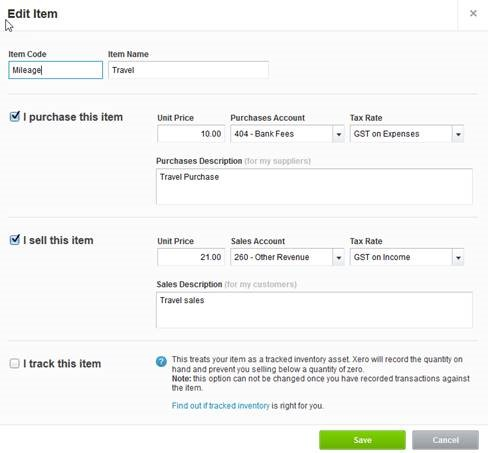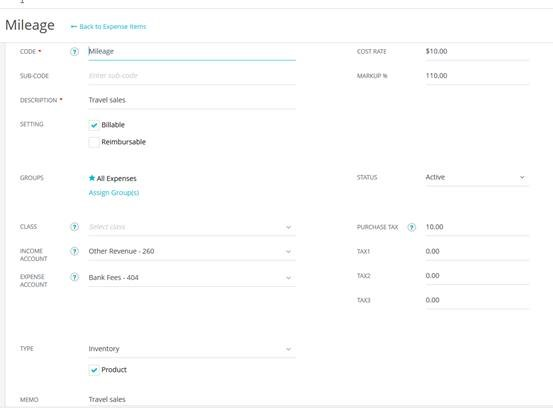## How do Xero expenses sync with CORE?

CORE expenses sync with Xero expenses. Here we are explaining the expense data flow between CORE and Xero with an example.

Let us take an expense item in Xero, Mileage Travel, with the following field values:As you can see, the Purchase Unit Price = 10

Sales Unit Price = 21

On syncing this item from Xero to CORE, the data is mapped in the following way in CORE:Xero Data Flow CORE Item Code <-> Expense Item Code Sales Description <-> Expense Item Description and Activity Item Memo Sales Unit Price <-> Markup Purchases Unit Price <-> Cost Rate Sales Account <-> Income Account Purchase Account <-> Expense Account Purchase Tax Code Default Rate <-> Purchase Tax Rate

From the above mapping, it is clear that:

• In this case, GST on Expenses tax code default rate is 10% in Xero and the Purchase Tax Rate for the synced expense in CORE is 10.
• Xero Sales Unit Price maps to the Markup in CORE by a calculation. To calculate the markup for the synced expense item in CORE, the formula is specified as below:

Markup = ((Sales Unit Price – Purchase Unit Price) / Purchase Unit Price) x 100

So, in the above case,

[(21 -10) / 10)] x 100 = 110

Accordingly, the markup in CORE is calculated as 110. So on syncing the specified expense item, Cost Rate will be 10, Purchase Tax Rate will be 10 (or any other value from the tax code in Xero) and markup will be 110.